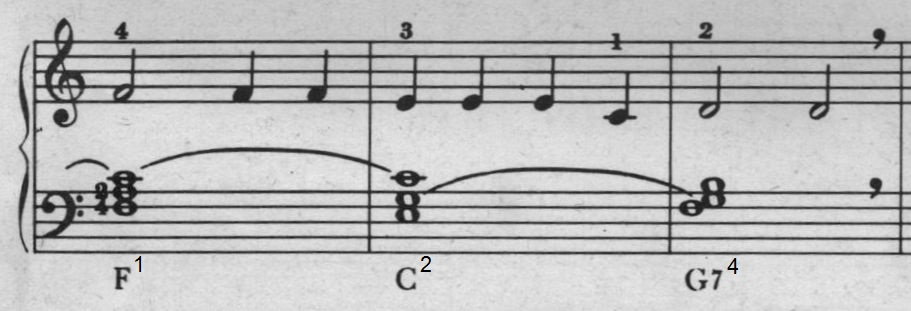## Is it still possible for mathematicians to contribute to the theory of music?

Key reference:
That set theory is useful with digital music, especially MIDI, seems not to be widely known.
Consider a sequence consisting of the following chords: $F^1 , C^2 , G7^4$ .Changing the chords means that the set of notes $F^1 = \{F,A,C\}$ is replaced by the set of notes $C^2 = \{E,G,C\}$ is replaced by the set of notes $G7^4 = \{F,G,B\}$ . Mind the notes in common; these are joined by bows in the score.
In MIDI, first the notes in $F^1$ are set On (Note On). After a duration of four beats, the notes in $C^2$ should sound. In order to accomplish smooth transition of the chords, this should be done by first hitting the chord $C^2$ before (immediately) releasing the chord $F^1$. More in detail, apply a Note On event to the elements in $\{E,G,C\}$ minus $\{F,A,C\} = \{E,G\}$ immediately followed (timestep $0$) by a Note Off event applied to the elements in $\{F,A,C\}$ minus $\{E,G,C\} = \{F,A\}$ . Note that nothing happens with the note $C$ .
After a duration of four beats again, the notes in $G7^4$ should sound. This should be done by first hitting the chord $G7^4$ before (immediately) releasing the chord $C^2$. More in detail, apply a Note On event to the members of the set $G7^4 \setminus C^2$ immediately followed by a Note Off event applied to the members of the set $C^2 \setminus G7^4$ .
This is in a nutshell how chord transition works - or rather should work - in MIDI. It's implemented in my personal mathematical contribution to music : MidiDoos .